Background
We begin with a slightly modified version of Equation (33.3) defining the discrete TR model: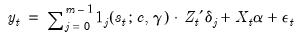(36.1)
where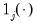is a (0, 1) indicator for regime that depends on the observed variable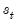, where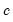represents one or more thresholds and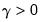is a threshold slope parameter. Note that we have divided the regressors into two groups—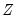variables whose coefficients vary across the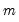regimes, and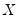variables with coefficients that are regime invariant.
Restricting ourselves to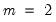, as using the fact that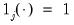for exactly one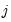, we may rewrite the discrete TR equation as: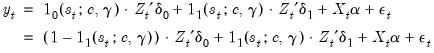(36.2)
To construct the two-regime STR model, we replace the indicator function with a continuous transition function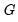that returns values between 0 to 1. Then we have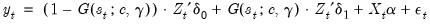(36.3)
wherehas different properties as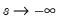,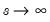, and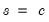, depending on the specific functional form.
The key modeling choices in Equation (36.3) are the choice of the threshold variable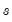and the selection of a transition function. For a givenand, we may estimate the regression parameters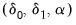and the threshold values and slope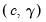via nonlinear least squares. Additionally, given a list of candidate variables for, we can select a threshold variable using model selection techniques.
Common transition function choices are given by:
Logistic (LSTR):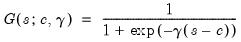(36.4)
Normal (NSTR or STR):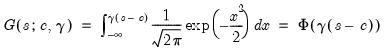(36.5)
Exponential (ESTR):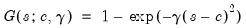(36.6)
Logistic, second-order (L2STR):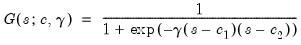(36.7)
forin all cases.
The logistic and normal transition functions are monotonically increasing inso that the two regimes correspond to high and low values of the threshold variable. The threshold valuedetermines the point at which the regimes are equally weighted, while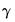controls the speed and smoothness of the transition. As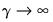, the transition function approaches the indicator function and the model approaches the discrete threshold model.
The exponential transition function is increasing in absolute deviations offrom the threshold. Furthermore,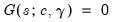when, andapproaches 1 asand. The ESTR model does not nest the discrete TR model since, as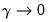or, the specification becomes linear sinceapproaches a constant function returning 0 or 1.
The second-order logistic function does nest the discrete TR model. Aswith distinct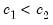,approaches 1 for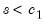and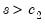, andapproaches 0 forin-between. Thus, the L2STR model nests a three-regime discrete TR model where the outer regimes have a common linear specification.
For finite,attains its minimum value at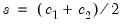with a non-zero value.
For further discussion of the implications of various choices for, see van Dijk, Teräsvirta, and Franses (2002) who offer extensive commentary on the properties of these transition functions and provide concrete examples of their use in empirical settings.
Following estimation of the coefficients using nonlinear least squares (van Dijk, TerasvirtTeräsvirta, and Franses, 2002; Leybourne, Newbold and Vougas, 1998), we may estimate the coefficient covariance matrix using conventional methods available for nonlinear least squares (ordinary, heteroskedasticity consistent, heteroskedasticity and autocorrelation consistent).
The general form of the NLLS covariance is given by: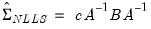(36.8)
where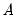is an estimate of the information,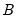is the variance of the residual weighted gradients, andis a scale parameter.
For the ordinary covariance estimator, we assume that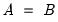. Then we have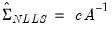(36.9)
whereis an estimator of the residual variance (with or without degree-of-freedom correction).
We may estimateusing the outer-product of the gradients (OPG) of the error function or the one-half of the Hessian matrix of second derivatives of the sum-of-squares function: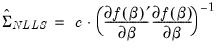(36.10)
or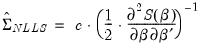(36.11)
evaluated at the estimated coefficients.
Alternately, we may assume distinctand, and employ a White or HAC sandwich estimator for the coefficient covariance as in “Robust Standard Errors”.
Lastly, following specification and estimation of an STR equation, the modeling cycle typically involves performing a number of hypothesis tests using the STR specification and results. Background information for the various tests performed by EViews will be discussed below.